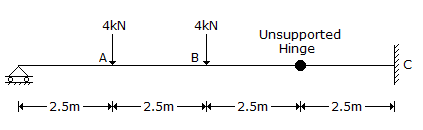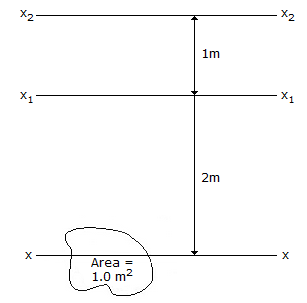# Civil Engineering - UPSC Civil Service Exam Questions

31.

The bending moments at point A, B, and C of the beam shown in the given figure will be:A. 10 kNm, 10 kNm and 10 kNm B. 10 kNm, 10 kNm and - 10 kNm C. 20 kNm, 10 kNm and - 10 kNm D. 10 kNm, - 10 kNm and 20 kNm

Explanation:

No answer description available for this question. Let us discuss.

32.

xx, x1 x1 and x2 x2 are parallel axes of which XX is the centroidal axis. If moment of inertia of the figure about x1 x1 axis is 10m4, what is the moment of inertia of the figure about x2 x2 axis?A. 10 m4 B. 11 m4 C. 14 m4 D. 15 m4

Explanation:

No answer description available for this question. Let us discuss.

33.

As per Lacey's regime equation, what is the flow velocity proportional to ?

 A. (Qf2)1/3 B. (Qf2)1/6 C. Q/f2 D. (Q/f)1/6

Explanation:

No answer description available for this question. Let us discuss.

34.

Which of the following types of pumps can be used for concreting ?
1. Piston operated.
2. Pneumatically operated.
3. Centrifugal operated, with straight blades.
4. Screw type.

 A. 1 and 3 B. 1 and 2 C. 1, 3 and 4 D. 2 and 4

Explanation:

No answer description available for this question. Let us discuss.

35.

For medium silt where average grain size is 0.16 mm, Lackey's silt factor is likely to be

 A. 0.3 B. 0.45 C. 0.7 D. 1.32